# Gear wheels

Two gear wheels, which fit together have number of teeth z1=58 and z2=149. Calculate the speed of the first wheel, if the second wheel rotates 1232 revolutions per minute.

Result

n1 =  3165 1/min

#### Solution:Leave us a comment of example and its solution (i.e. if it is still somewhat unclear...):

Showing 0 comments:Be the first to comment!#### To solve this example are needed these knowledge from mathematics:

Need help calculate sum, simplify or multiply fractions? Try our fraction calculator. Do you want to convert length units?

## Next similar examples:

1. What isWhat is the value of the smaller of a pair of numbers for which their sum is 78 and their division quotients are 0.3?
2. Rotatable tower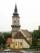Rotatable tower situated in the city center has ground shape of a regular polygon. If the tower is rotated by 14.4° around its centerpiece it looks from the side same. Your task is to calculate at least how many vertices can have a ground plan view of the.
3. Icecreams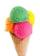Karthik bought 30 icecreams for his birthday .2/5 of them are chocolate icecreams. Find the number of chocolate icecreams he bought?
4. Ratio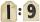Unfold number 1963 in the ratio 9:2:2.
5. Classroom 4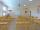In a class of 36 pupils, 2/3 are girls. How much it is in a class girls and boys?
6. Balls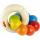The boys changed stamps, beads and balls. For 9 balls is 3 stamps, 2 balls is 44 stamps. How many beads is for 1 ball?
7. Medians 2:1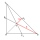Median to side b (tb) in triangle ABC is 12 cm long. a. What is the distance of the center of gravity T from the vertex B? b, Find the distance between T and the side b.
8. Women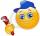In an company of 1050 employees are 2/3 women. How many women work in the company?
9. Unit rateFind unit rate: 6,840 customers in 45 days
10. Tallest and shortestJenn is the tallest on the team. He is 1 1/2 times as tall as the shortest girl whom is 4 1/4 feet tall. How tall is Jebb?
11. Product of two fractionsProduct of two fractions is 9 3/5 . If one of the fraction is 9 3/7. Find the other fraction.
12. Watching TVOne evening 2/3 students watch TV. Of those students, 3/8 watched a reality show. Of the students that watched the show, 1/4 of them recorded it. What fraction of the students watched and recorded reality tv.
13. AnglesIn the triangle ABC, the ratio of angles is: a:b = 4: 5. The angle c is 36°. How big are the angles a, b?
14. Scale of the mapDetermine the scale of the map if the actual distance between A and B is 720 km and distance on the map is 20 cm.
15. Farmers 2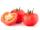On Wednesday the farmers at the Grant Farm picked 2 barrels of tomatoes. Thursday, the farmers picked 1/2 as many tomatoes as on Wednesday. How many barrels of tomatoes did the farmers pick on Thursday?
16. Ratio 11Simplify this ratio 10 : 1/4
17. Fractions 4How many 2/3s are in 6?